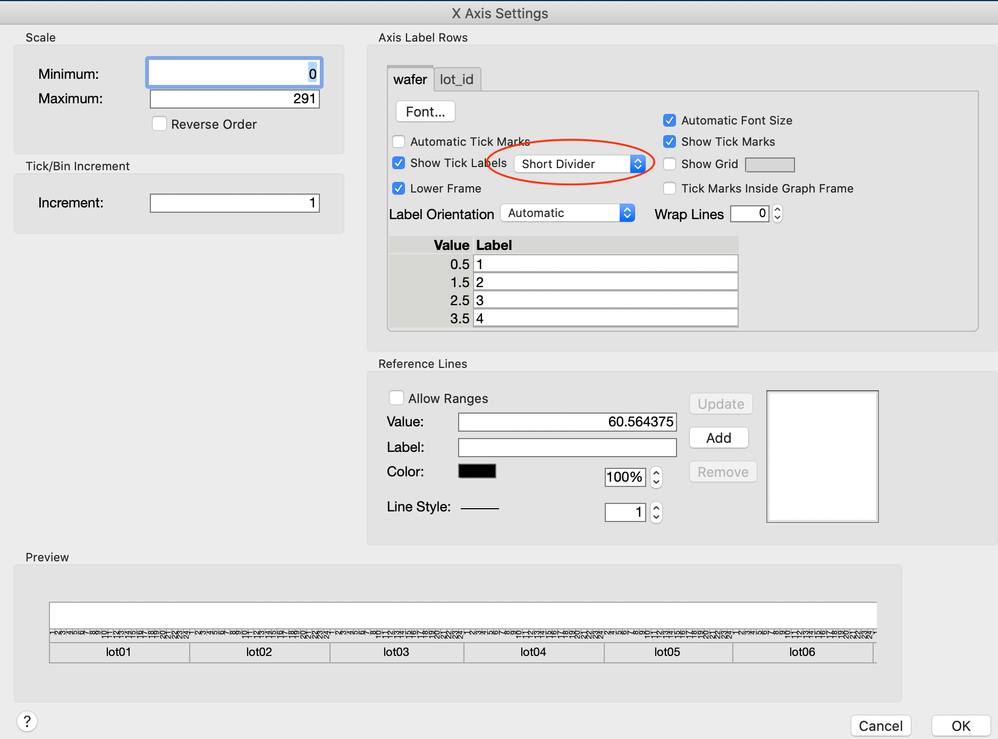Our World Statistics Day conversations have been a great reminder of how much statistics can inform our lives. Do you have an example of how statistics has made a difference in your life? Share your story with the Community!
Choose Language Hide Translation Bar
Highlighted

## Whitespaces in plot labels

Hi all,

How can I remove the whitespaces in my plot labels? I'm using this function to plot

``````varplot3 = Function( { y_param },
var = Variability Chart(
Y( column( y_param ) ),
X( evallist( varlist2 ) ),
Analysis Type( "Choose best analysis (EMS REML Bayesian)" ),
Mean Diamonds( 0 ), Points Jittered( 0 ), Connect Cell Means( 1 ),
Show Box Plots( 0 ), Std Dev Chart( 0 ) );
rvar = var << Report;
rvar[Axisbox( 1 )] << Show Major Grid( 1 );
rvar[Framebox( 1 )] << {Marker Size( 4 )};
rvar[Framebox( 1 )] << Frame Size(700, 300);
rvar[TextEditBox( 1 )] << Set Font Size( 10 ) << Set Font Style( "Bold" );
rvar[NomAxisBox( 4 )] << {Rotated Tick Labels( 0 )};
rvar[Framebox( 1 )] << Row Legend( Column( buildname ), Color( 1 ), Marker( 0 ), Color Theme("JMP Default"), Continuous Scale( 0 ) );
rvar[Framebox( 1 )] << Row Legend( Column( ht ), Color( 0 ), Marker( 1 ), Marker Theme("Paired") );
g = nitems(varlist2)+2;
Try(rvar[BorderBox(g+1)] << Delete);
Try(rvar[BorderBox(g)] << Delete);
pvar = rvar[Outlinebox( 2 )] << get picture;
);``````

I also attached the sample output that does have whitespaces in the label. How to remove the whitespaces? PLs help

3 REPLIES 3
Highlighted

## Re: Whitespaces in plot labels

Try this. Make the plot, then right click on the axis to bing up 'Axis Settings...'. Then select 'Short Divider':If this is what you are after, save the script and steal the extra code you need. You will see something like:

``````		Dispatch(
{"Variability Chart for NPN1"},
"1",
ScaleBox,
{Label Row( 1, Tick Mark Style( "Short Divider" ) )}
)``````

Highlighted

## Re: Whitespaces in plot labels

Hi, I tried inserting the line into my function but it still doesn't work.

``````rvar = var << Report;
rvar[ScaleBox( 1 )] << {Label Row( 1, Tick Mark Style( "Short Divider" ) )};
rvar[Axisbox( 1 )] << Show Major Grid( 1 );
rvar[Framebox( 1 )] << {Marker Size( 4 )};
rvar[Framebox( 1 )] << Frame Size( 700, 300 );
rvar[Text Edit Box( 1 )] << Set Font Size( 10 ) << Set Font Style( "Bold" );
rvar[NomAxisBox( 4 )] << {Rotated Tick Labels( 0 )};
rvar[Framebox( 1 )] << Row Legend(
Column( buildname ),
Color( 1 ),
Marker( 0 ),
Color Theme( "JMP Default" ),
Continuous Scale( 0 )
);
rvar[Framebox( 1 )] << Row Legend( Column( ht ), Color( 0 ), Marker( 1 ), Marker Theme( "Paired" ) );``````
Highlighted

## Re: Whitespaces in plot labels

Hi,
I don't think you need the list brackets { }.
Best,
TS
Thierry R. Sornasse
Article Labels